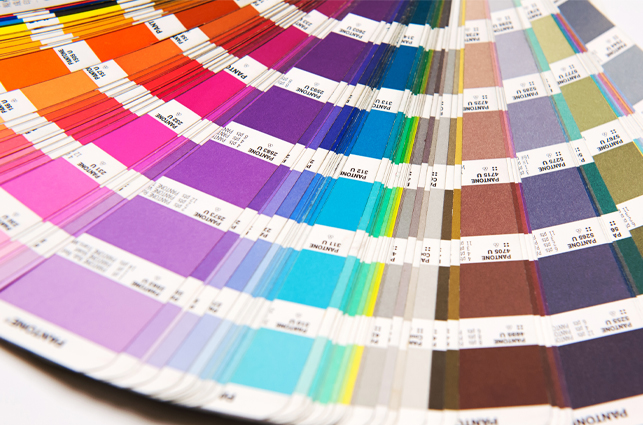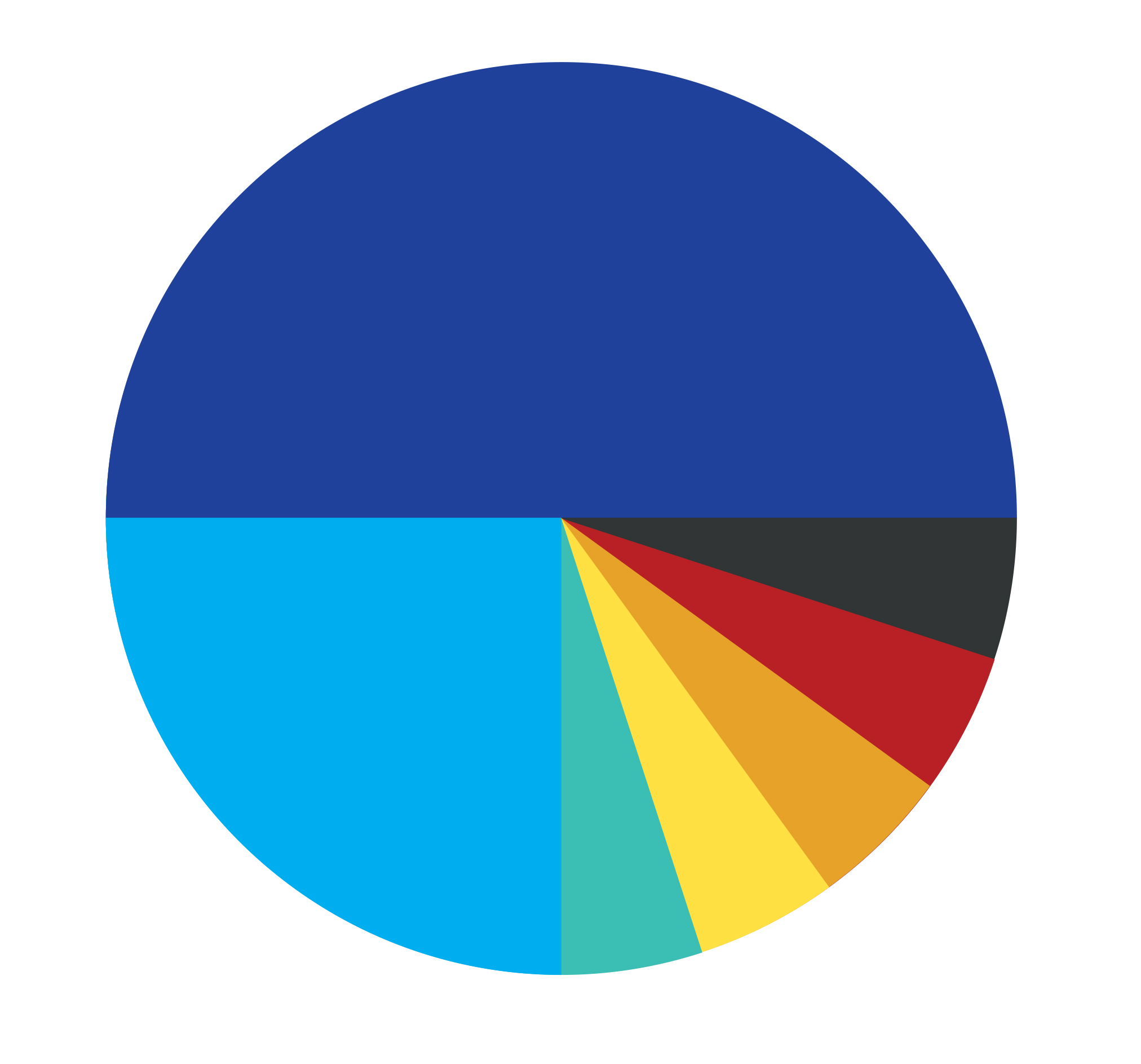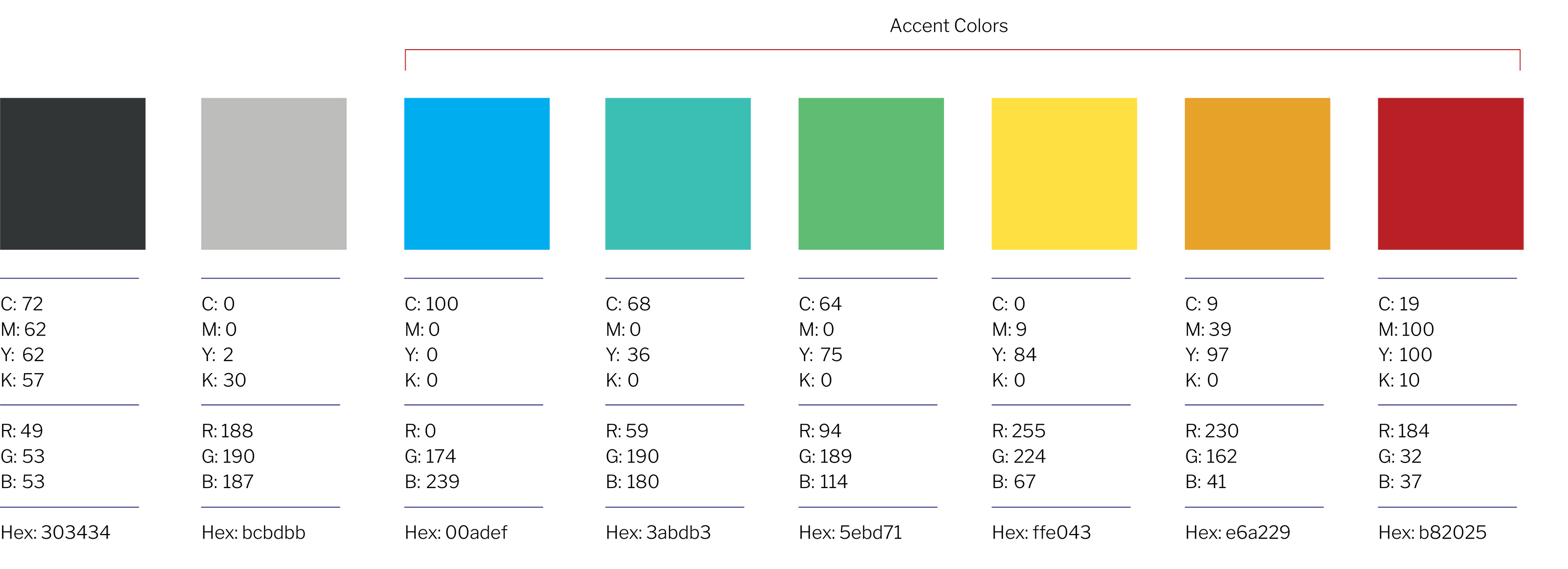# Color Palette

El Camino’s primary color is El Camino Blue (#20419A) and should be used at 100 percent opacity.

## Primary Color

The primary El Camino College color is blue as specified here. Blue is the color which should be the color El Camino is recognized by.El Camino Blue C=100 M=89 Y=0 K=0R=32 G=65 B=154#20419aPMS 661

## Secondary Colors

Shown below are accent colors which can be used in a conservative secondary role and should be used to a much lesser degree than the El Camino Blue.C=72 M=62 Y=62 K=57R=49 G=53 B=53#303434C=0 M=0 Y=2 K=30R=188 G=190 B=187#bcbdbbC=100 M=0 Y=0 K=0R=0 G=174 B=239#00adefC=68 M=0 Y=36 K=0R=59 G=190 B=180#3abdb3C=64 M=0 Y=75 K=0R=94 G=189 B=114#5ebd71C=0 M=9 Y=84 K=0R=255 G=224 B=67#ffe043C=9 M=39 Y=97 K=0R=230 G=162 B=41#e6a229C=19 M=100 Y=100 K=10R=184 G=32 B=37#b82025

### Color DistributionThe color wheel illustrates the weight which should be given to the secondary colors.

The secondary colors should be used only to add energy and interest to marketing materials and not compete with El Camino Blue.

The secondary color palette was designed to complement the overall look and feel of the El Camino's brand. These colors should be used only in a supporting manner to the primary brand colors.

The color wheel illustrates the weight which should be given to the secondary colors.